Fractions - math word problems - page 6

1. Forest nursery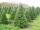In Forest nursery plant one pine to 1.9 m2. Calculate how many plants are planting in area 362 acres.
2. Brick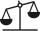Isosceles scale has on one side all brick and second weight 1 kg and 1/4 of brick. The balance is in equilibrium. What is the weight of a brick?
3. Disinfecting solutionHow much distilled water is necessary to pour into 500 ml of 33% hydrogen peroxide solution to obtain 3% disinfecting solution?
4. Minute hand v2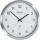In how many minutes describe the minute hand angle 60 degrees?
5. Class 8.AThree quarters of class 8.A went skiing. Of those who remained at home one third was ill and the remaining six were on math olympic. How many students have class 8.A?
6. Self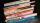A math text book is 2 2/9 inches thick. how many of these books will fit on a 120-inch self?
7. Soup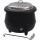On Monday we cook 25 pots and 10 boilers of soup. On Tuesday 15 pots and 13 boilers. On Wednesday 20 pots and on Thursday 30 boilers. On Monday and Tuesday was cooked the same amount of soup. How many times more soup cooked on Thursday than on Wednesday?
8. Ray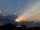Light ray loses 1/19 of brightness passing through glass plate. What is the brightness of the ray after passing through 7 identical plates?
9. Clock hands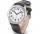The hands on the clock shows the time 12 hours and 2 minutes. Calculate the size of a sharp angle between clock hands three hours later.
10. Tree trunk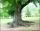Calculate the average tree trunk with a circumference of 149 cm.
11. FractionFraction ? write as fraction a/b, a, b is integers numerator/denominator.
12. Alloy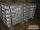The first alloy is a mixture of two metals in the ratio 1:2, the second is a mixture of same metals in the ratio 2:3. At what ratio we have these two alloys put into the furnace to obtain a new metal alloy with ratio 17:27? (All three ratios correspond to.
13. TV commercials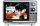For the typical one-hour prime-time television slot, the number of minutes of commercials is 3/8 of the minutes of the actual program. Determine how many minutes of the program are shown in that one hour.
14. FractionDetermine for what x fraction ?:
15. Equation with fractionsSolve equation: ? It is equation with fractions.
16. Pocket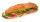Mirka spent on a trip for gifts half pocket. For the third remain money bought a bagel. Six euros left her. How many euros had Mirka in pocket?
17. BevelI have bevel in the ratio 1:6. What is the angle and how do I calculate it?
18. Scale of the mapDetermine the scale of the map, which is the actual distance of 120 km l represented by segment long 6 cm.
19. Homework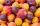In the crate are 18 plums, 27 apricot and 36 nuts. How many pieces of fruit left in the crate when Peter took 8 ninth: 1. nuts 2. apricots 3. fruit 4. drupe
20. Cups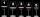We have three cups. In the cups we had fluid and boredom we started to shed. 1 We shed one-third of the fluid from the second glass into the first and third. 2 Then we shed one quarter cup of liquid from the first to the second and to the third. 3 Then

Do you have an interesting mathematical word problem that you can't solve it? Enter it, and we can try to solve it.

To this e-mail address, we will reply solution; solved examples are also published here. Please enter e-mail correctly and check whether you don't have a full mailbox.

Need help calculate sum, simplify or multiply fractions? Try our fraction calculator.